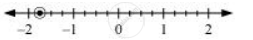Share

# Draw the Number Line and Represent the Following Rational Numbers on It] (-7)/4 - CBSE Class 7 - Mathematics

ConceptRational Numbers Between Two Rational Numbers

#### Question

Draw the number line and represent the following rational numbers on it]

(-7)/4

#### Solution

(-7)/4 = -1 3/4

This fraction represents 1 full part and 3 parts out of 4 equal parts. Negative sign represents that it is on the negative side of the number. Therefore, each space between two integers on number line must be divided into 4 equal parts.

(-7)/4can be represented asIs there an error in this question or solution?

#### APPEARS IN

NCERT Solution for Mathematics for Class 7 (2018 to Current)
Chapter 9: Rational Numbers
Ex. 9.10 | Q: 4.3 | Page no. 183
Solution Draw the Number Line and Represent the Following Rational Numbers on It] (-7)/4 Concept: Rational Numbers Between Two Rational Numbers.
S